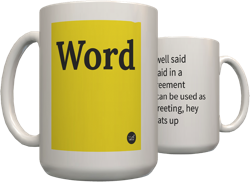Top definition
Back in the 90's when pagers were made available to a younger generation, a code was created where numbers were assigned to represent letters so that messages could be passed using this code.

Each letter was represented using a number or combination of numbers. Some number definitions varied but this is just one basic breakdown:

A = 8
B = 8
C = 6
D = 0
E = 3
F = 4
G = 6
H = 4
I = 1
J = 7
K = 15
L = 7
M = 177
N = 17
O = 0
P = 9
Q = 0
R = 12
S = 5
T = 7
U = 11
V = 11
W = 111
X = 25
Y = 4
Z = 2

There was often a lot of confusion with messages.
7415 15 986312 6003 81764
( This is pager code bitch )
by Alex Cho December 22, 2004Get a pager code mug for your bunkmate Trump.
2
Pager codes were used to type out letters using numbers back in the day when pagers were popular (before cellphones became mainstream). You would do this WITHOUT having to flip your pager upside down. A=6 B=8 C=0 D=0 E=3 F=7 G=9 H=4 I=1 J=7 K=15 L=7 M=177 N=17 O=0 P=9 Q=9 R=12 S=5 T=7 U=17(or 11) V=17(or 11) W=111 X=25 Y=4 Z=5. They are made to look as close to the actual letter as possible (Keep in mind that the font used on pagers show the numbers differently then this font). It's a little confusing at first, and takes you longer to figure out a word because some numbers stand for the same letters, but if you get a few of the letters, you can usually figure it out.
969312 00035 1113123 50 17537177 860153 117 743 064." "PAGER CODES WERE SO USEFUL BACK IN TH DAY.
by jezzzzka75 June 04, 2010Get a pager code mug for your brother-in-law Manley.
buy the domain for your travel vlog